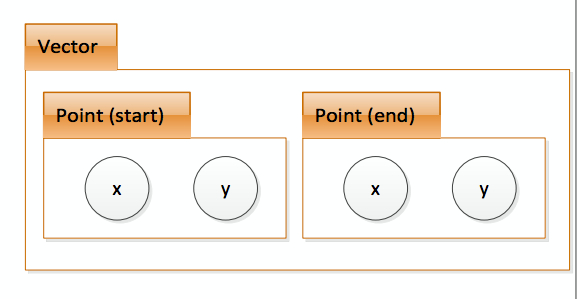# Erle Robotics C++ GitBook

## Classes of classes

As we have explained in the beginning example:a point consists of an x and y coordinate and a vector consists of 2 points: a start and a finish. So the scheme is the following:class Point {
public:
double x;
double y;
};
class Vector {
public:
Point start;
Point end;
};


So fields can be classes. Note the order in which classes are defined. To access the fields we should do the following:

class Point {
public:
double x, y;
};

class Vector {
public:
Point start, end;
};

int main() {
Vector vec1;// define vec1 of Vector class
vec1.start.x = 3.0;
vec1.start.y = 4.0;
vec1.end.x = 5.0;
vec1.end.y = 6.0;
}


You can also assign one instance to another, that means copy all fields.

vec2.start = vec1.start;